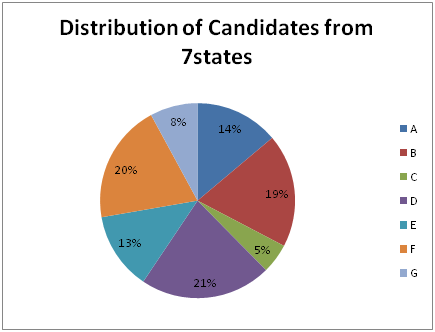# Common problem asked in Data Interpretation

Today I'm going to discuss a very important question of Data interpretation. This type of question has also been asked so many times in Quantitative Aptitude section. Read the question and try to solve it yourself first.

Problem: Study the graph and try to answer the questions.Total candidates appeared = 2 lakh

Table: State-wise percentage and ratio of Male and Female Qualified candidates

 State Per cent Qualified Ratio of qualified candidates Male Female A 50 4 3 B 60 6 2 C 55 3 2 D 45 2 1 E 65 5 4 F 55 6 5 G 50 2 6

1. Find total number of females qualified from states C and D together.

2. Find number of male candidates qualified from state B.

3. Which of the following pair of states have equal number of qualified male candidates?
a) C,G    b) B,F    c) C,E    d) None of these.

4. What is the total number of candidates qualified from states F and G together?

Solution: Total number of candidates = 2 lakh

Study the above chart and table first and calculate the things you require to solve the questions just as follows:

 States %age Of Candidates(from pie chart) Number of candidates (2lakh*percentage) Qualified Candidates Male: Female Male Female A 14 28,000 14,000(50%) 4:3 8,000 6,000 B 19 38,000 22,800(60%) 6:2 17,100 5,700 C 5 10,000 55,00(55%) 3:2 33,00 22,00 D 21 42,000 18,900(45%) 2:1 12,600 6,300 E 13 36,000 23,400(65%) 5:4 13,000 10,400 F 20 40,000 22,000(55%) 6:5 12,000 10,000 G 8 16,000 8,000(50%) 2:6 2,000 6,000

For A = Candidate appeared = 14% of 2,00,000 = 28,000

Out of 28,000 50% qualifies the exam = 50% of 28,000 = 14,000

Now, ratio of male and female = 4:3
Therefore, number of males qualified = (14,000) times 4/7 = 8,000

Number of females qualified = 14,000 - 8,000 = 6,000

Similarly I calculate for others. Now using this table, we can easily answer the questions

1. Total number of females qualified from states C and D together = 2200 + 6300
⇒8500

2. Number of male candidates qualified from state B = 17100

3. No two states have equal number of qualified male candidates.

4. Total number of candidates qualified from states F and G together = 22,000 + 8000
⇒30,000

Note:You don't need to make the above table. I just calculate everything to make you understand. Do the required calculations only.

Check the other posts of Data interpretation also

Data Interpretation: Tables
Data Interpretation: Bar-charts